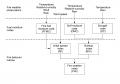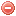# Description

The Canadian forest fire weather index ($$FWI$$) system was developed in Canada in the 1970s based on several decades of forest fire research. It was modified or improved several times since then (Van Wagner 1987).

This fire weather index system consists of six standard components based on weather data and date (month) only: three primary fuel moisture indices ($$FFMC$$, $$DMC$$, $$DC$$), two intermediate fire behavior indices ($$ISI$$ and $$BUI$$), and a final fire intensity index ($$FWI$$) resulting from the combination of the preceding sub-indices (Van Wagner 1987).

The $$FWI$$ system is based on weather data only. The different sub-indices require noon temperature, precipitation, relative humidity, and/or wind speed as input data. Although the weather variables have to be measured at noon, the indices represent fire danger at mid-afternoon (around 16:00).

The $$FWI$$ system and its components have been found to be suitable for reflecting various aspects of fire activity (Van Wagner 1987): in particular, the $$FFMC$$ correlates well with fire occurrence; the $$ISI$$ with burnt area; and the $$BUI$$ and $$FWI$$ with fire activity in general.

Although these indices were primarily developed based on fuel types and fire behavior data from pine forests, they were designed for predicting fire danger throughout Canada (Van Wagner 1987).

The FWI system is also widely used, with or without adaptation to local conditions, in other regions or countries than Canada, such as Alaska, some northern American states, Mexico, New Zealand, France, Portugal or Southeast Asia.

# Structure

The first level of the FWI system consists of three fuel moisture codes. These codes reflect the moisture content of different type of fuels (Van Wagner 1987):
• the Fine fuel moisture code ($$FFMC$$), which represents the moisture content of litter and fine fuels, and requires temperature, relative humidity, wind speed and precipitation as input data
• the Duff moisture code ($$DMC$$), which represents the moisture content of loosely compacted, decomposing organic matter, and requires temperature, relative humidity and precipitation as input data
• the Drought code ($$DC$$), which represent the moisture content of deep compact layer of organic matter, and requires temperature and precipitation as input data

The second level of the FWI system consists of three fire behavior indices (Van Wagner 1987):
• the Initial spread index ($$ISI$$), which combines the $$FFMC$$ and wind speed, and represents the rate of fire spread
• the Buildup index ($$BUI$$), which combines the $$DMC$$ and the $$DC$$, and represents the total fuel available to the spreading fire
• the Fire weather index ($$FWI$$), which combines the $$ISI$$ and the $$BUI$$, and represents fire intensityFigure 1. Structure of the FWI system (sources: Van Wagner 1987)

# Index interpretation

cf. $$FWI$$

Latitude has an influence on day length and thereby on the amount of drying which can occur on a given day. The $$DMC$$ and $$DC$$ include a day length factor which changes month by month, and is constant over the whole Canada (Van Wagner 1987).

These day length factors are valid for one latitude only (about 46° N in Canada), and are therefore not suitable for regions at other latitudes. Accordingly, adjustments of these day length factors have been undertaken in some countries in order to allow for the use of the FWI system at different latitudes, e.g. in New Zealand and Southeast Asia (cf. Alexander 1994 and de Groot et al. 2007), than that at which it had originally been developed (Lawson & Armitage 2008). In Lawson & Armitage (2008), standardized day length adjustment factors for the $$DMC$$ and $$DC$$ at different latitudes are proposed. NB: European countries should use Canadian standard values.

Original publication:
An extensive literature about the FWI system and its different components is available. For a detailed description, see Van Wagner (1987).

Other publications:
Alexander (1994)
de Groot et al. (2007)
Lawson et Armitage (2008)

### Bibliography SearchNo results!

No results were found. Are you sure you searched for a tag?

### Symbols

 Variable Description Unit $$T$$ air temperature °C $$T_{dew}$$ dew point temperature °C $$H$$ air humidity % $$P$$ rainfall mm $$U$$ windspeed m/s $$w$$ days since last rain (or rain above threshold) d $$rr$$ days with consecutive rain d $$\Delta t$$ time increment d $$\Delta{e}$$ vapor pressure deficit kPa $$e_s$$ saturation vapor pressure kPa $$e_a$$ actual vapor pressure kPa $$p_{atm}$$ atmospheric pressure kPa $$PET$$ potential evapotranspiration mm/d $$r$$ soil water reserve mm $$r_s$$ surface water reserve mm $$EMC$$ equilibrium moisture content % $$DF$$ drought factor - $$N$$ daylight hours hr $$D$$ weighted 24-hr average moisture condition hr $$\omega$$ sunset hour angle rad $$\delta$$ solar declination rad $$\varphi$$ latitude rad $$Cc$$ cloud cover Okta $$J$$ day of the year (1..365/366) - $$I$$ heat index - $$R_n$$ net radiation MJ⋅m-2⋅d-1 $$R_a$$ daily extraterrestrial radiation MJ⋅m-2⋅d-1 $$R_s$$ solar radiation MJ⋅m-2⋅d-1 $$R_{so}$$ clear-sky solar radiation MJ⋅m-2⋅d-1 $$R_{ns}$$ net shortwave radiation MJ⋅m-2⋅d-1 $$R_{nl}$$ net longwave radiation MJ⋅m-2⋅d-1 $$\lambda$$ latent heat of vaporization MJ/kg $$z$$ elevation m a.s.l. $$d_r$$ inverse relative distance Earth-Sun - $$\alpha$$ albedo or canopy reflection coefficient - $$\Delta$$ slope of the saturation vapor pressure curve kPa/°C $$Cc$$ cloud cover eights $$ROS$$ rate of spread m/h $$RSF$$ rate of spread factor - $$WF$$ wind factor - $$WRF$$ water reserve factor - $$FH$$ false relative humidity - $$FAF$$ fuel availability factor - $$PC$$ phenological coefficient -

 Suffix Description $$-$$ mean / daily value $$_{max}$$ maximum value $$_{min}$$ minimum value $$_{12}$$ value at 12:00 $$_{13}$$ value at 13:00 $$_{15}$$ value at 15:00 $$_{m}$$ montly value $$_{y}$$ yearly value $$_{f/a}$$ value at fuel-atmosphere interface $$_{dur}$$ duration $$_{soil}$$ value at soil level

 Constant Description $$e$$ Euler's number $$\gamma$$ psychrometric constant $$G_{SC}$$ solar constant $$\sigma$$ Stefan-Bolzmann constant